Ecological Archives E094-241-A4

Junji Konuma, Teiji Sota, Satoshi Chiba. 2013. A maladaptive intermediate form: a strong trade-off revealed by hybrids between two forms of a snail-feeding beetle. Ecology 94:2638–2644. http://dx.doi.org/10.1890/12-2041.1

Appendix D. Statistical significance of the trade-off.

To confirm the statistical significance of the strength of the trade-off, we used the method proposed by O’Hara Hines et al. (2004). Their method assumes the null hypothesis that the fitness of the intermediate phenotype is collinear with those of the divergent phenotypes. This corresponds to the situation in which the feeding performance of the intermediate beetle phenotype is located somewhere along a straight line that connects the performance of the stout and slender phenotypes (e.g., line 2 in Fig. 1A). The alternative hypothesis is that the intermediate phenotype has a higher or lower fitness than the average fitness of the divergent phenotypes, such that the feeding performance of the intermediate phenotype is located along line (1) or lines (3) and (4) in Fig. 1A. According to O’Hara Hines et al. (2004), a test statistic L is the sum of the following components lij: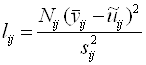,

where i = 1, 2, and 3 represent the stout, intermediate, and slender phenotypes, respectively; j = 1 and 2 represent experimental conditions with large and small snails, respectively; Nijis the sample size;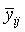is the average feeding success calculated using raw data;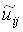is the estimated mean that is calculated using the equations in appendix B of O’Hara Hines et al. (2004); and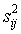is the variance of feeding success. Data sets for beetle populations P1, F1, and P2 were used as i = 1, 2, and 3, respectively.

O’Hara Hines et al. (2004) suggest that a chi-square test would be effective for calculating P values in experimental designs with binominal-type data, such as “dead or alive” and “success or failure”. A chi-square test is generally used to assess the goodness of fit, in which the test statistic is the sum of the differences in each independent event between observed and expected values. If observed data match the null hypothesis, the test statistic is close to 0, whereas the null hypothesis is rejected with large values of the test statistic; note that the test statistic can never have a negative value. In the method of O’Hara Hines et al. (2004),andcorrespond to the observed and expected values, respectively. The distribution of the test statistic lij is approximately close to a chi-square distribution with 1 df (Kalbfleisch 1985), and O’Hara Hines et al. (2004) confirmed that a chi-square test is robust and statistically powerful for binominal-type data from a simulation study (Appendix C in O’Hara Hines et al. 2004).

A summary of the test statistics is given in Table D1. Although observed () and expected values () were different in the five pairings of i (phenotype) and j (experimental conditions), both were zero for the test with slender beetles on small snails because of a complete failure in feeding. Therefore, when obtaining the test statistic L by summing up lij, we cannot include l3,2 for slender beetles on small snails. However, because lij is > 0 by definition and larger values of L will be more significant, we can proceed with determining if the null hypothesis can be rejected without accounting for the component l3,2. The summed value L was 3.63, which corresponds to a one-sided P value = 0.028 (df = 1), and the true P would not be larger than this value. Thus, we can say that the trade-off curve bends inward and represents a strong trade-off.

Table D1. Summary of the test statistics suggested by O’Hara Hines et al. (2004).

 Stout shape Intermediate shape Slender shape Large snail Small snail Large snail Small snail Large snail Small snail Sample size (Nij) 24 24 24 24 24 24 Observed values (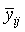) 0.25 0.63 0.17 0.25 0.46 0.00 Expected values (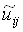) 0.18 0.60 0.27 0.29 0.36 0.00 SD (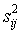) 0.20 0.24 0.14 0.20 0.26 0.00 Test statistic components (lij) 0.60 0.07 1.86 0.23 0.86 NA

NA, not available.

LITERATURE CITED

O’Hara Hines, R. J., and W. G. S. Hines, and B. W. Robinson. 2004. A new statistical test of fitness set data from reciprocal transplant experiments involving intermediate phenotypes. American Naturalist 163: 97–104.

Kalbfleisch, J. G. 1985. Probability and statistical inference. Vol. 2. Second edition. Springer, New York, New York, USA.